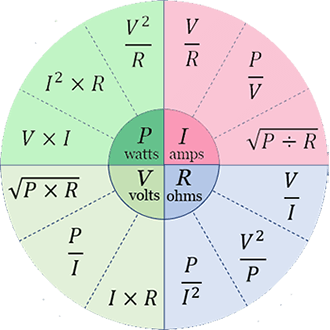# Ohm’s law calculator

### Ohm's Law Calculator

Volts to Watts Calculator >>

One of physics’ fundamental rules is Ohm’s Law. This section explains the relationship between voltage, current, and resistance. In an electrical field, voltage is the potential difference between two locations. The movement of electrical charge carriers, such as electrons or electron-deficient atoms, is referred to as amperage. A particle’s resistance to electric current flow is indicated by the last word, resistance.

According to Ohm’s law, the voltage and current in an electrical device have a linear relation.

The voltage drop and resistance of the resistor determine the DC current flow across the resistor.

Using a water flow analogies, we may consider the electric current as a water current flowing through the pipe, the resistors as a narrow pipe limiting the flow of water, and the volts as a vertical distance in the water allowing the water to flow.

Similarly, many materials are conductors and resistors across a wide range of voltages and currents, so the conductance and resistance of electronic components made from some of these materials will remain unchanged.

No matter whether the driving voltage or current vary over time or not, Ohm’s Law holds true for circuits with only resistive components.

There are a variety of equations that can be used to calculate it, but the most common one is the combination of the three.

I = V ÷ R

P = V * I

V = I * R

R = V ÷ I

### Electrical Power

It is a measure of how much electrical energy can be transported per unit time by an electric circuit and is known as power in the SI units.

Electric generators provide electricity, which is then distributed to companies and residences through the electric power sector, although it can also come from electric batteries or other sources.

Joule’s Law may be used with Ohm’s Law in resistor circuits to get various formulations for the amount of power dissipated, as demonstrated below.

P = V * I

P = V² ÷ R

P = I² * R

Where,

V = voltage
R = resistance
I = current
P = power

### Ohm’s Law Equation Wheel### Ohm’s law calculator for AC

To get the other values, enter two magnitudes plus the phase angle and press the Calculate button.

°
=
°
°
°
=

Volts to amps calculator >>

### Calculation formula for AC Ohm’s law

The current I in amps (A) times the impedance Z in ohms () equals the voltage V in volts (V).

V = I × Z = (|I|×|Z|) ∠ (θI + θZ)

In volt-amps (VA), the complicated power S.

S = (|V|×|I|) ∠ (θV – θI)